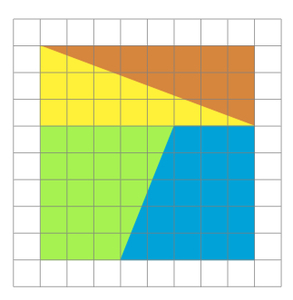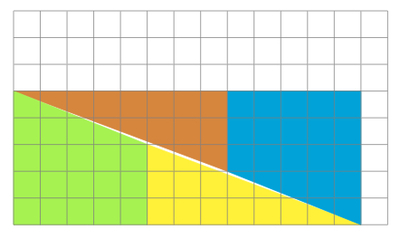# Different Areas?

Alignments to Content Standards: 8.G.A.4 8.EE.B

Below is a picture of an 8 by 8 square divided into four polygons:The green and yellow polygons can be combined along their 3 unit edges as shown below:1. What is the area of the green and yellow shape above?  What about the area of the blue quadrilateral and orange triangle?
2. What is the area of the original square?
3. Are the answers to (a) and (b) consistent? Explain.

## IM Commentary

The goal of this task is to motivate a discussion of similarity and slope via a counterintuitive geometric construction where it appears as if area is not conserved by cutting and reassembling a simple shape. There are many viable approaches to this task including:

• Putting together the two congruent right ''triangles'' to make a ''rectangle'' and observing that they do not fit properly, leaving a long thin empty space which explains the discrepancy in areas.
• Calculating the slopes of the two line segments on the quadrilateral which appears to be a triangle: they are not the same and this means that the shape is not actually a triangle.
• Applying a dilation to one of the 3 unit by 8 unit triangles and observing that it does not make a 5 by 13 triangle.

It is important for students to see explicit examples where visual reasoning can lead us astray. In this case, what appears to be a single line segment is in fact two line segments sharing a vertex. The slopes are close enough, however, that it is not easy to see this. It is also difficult to visually check if a given shape is, for example, a square, parallelogram, rectangle, or rhombus. In each case, a small change in an angle or side length (so small that it can not be detected visually) will take a shape out of these categories. As students move from the physical representations of side lengths and angles in elementary school toward the more abstract arguments of high school, it is important that they see examples which motivate the need for more precise models.

In part (a) students are not prompted to find the area of the combined triangle made by the green and yellow shapes as then the instruction would be deliberately misleading. If, however, students find the area of these two shapes separately and add them up then their answers to (a) and (b) will be consistent and part (c) will lose some of its interest. On the other hand, if different students take different approaches then this should provide for lively discussion.

The teacher may wish to make cut outs available for the four shapes making up the original square so that students can physically rearrange them and proceed to the area calculations. This will also allow them to reassemble the four pieces as in the solution to part (c). This has the added advantage of providing powerful visual evidence that the combined quadrilateral and triangle from part (a) is not in fact a triangle.

## Solution

1. If we calculate separately, the yellow triangle is 8 units by 3 units and so its area is 12 square units. The green quadrilateral can be decomposed into a 3 unit by 5 unit rectangle (of area 15 square units) and a 5 unit by 2 unit right triangle (of area 5 units). Adding these all up we get 32 square units.

On the other hand, the shapes when combined appear to form a 5 by 13 right triangle which would have area $\frac{65}{2}$ square units which is $\frac{1}{2}$ square units more than the previous calculation. This apparent paradox will be resolved in (c).

2. The square is 8 units by 8 units so its area is 64 square units.
3. If we calculate the areas of the four polygons which make up the square then these add up to the area of the square. On the other hand, the answers to 1 and 2 are not consistent if the green and yellow shapes make a triangle when put together as in the picture. So it must be that what appears to be a triangle is not a triangle. We can show that this is the case in a number of ways:

• Although it does not explain why the shape is not a triangle, the area calculations in parts a and b show that it cannot be a triangle because area is additive when shapes are divided and recombined without overlap (sharing only edges or vertices).
• We can find the slopes of the upper edges of the green quadrilateral and the yellow triangle. For the quadrilateral we move to the right 5 and down 2 so this is a slope of -$\frac{2}{5}$. For the triangle we move right 8 and down 3 for a slope of $-\frac{3}{8}$. These slopes are not the same and so the two segments combined do not make part of a line (any two distinct points on a non-vertical line give the same slope).
• The horizontal and vertical side lengths of the yellow triangle are 8 units and 3 units. The horizontal and vertical side lengths of the combined yellow and green shapes are 13 units and 5 units. If the large shape were  a triangle, it would be similar to the yellow triangle as both have a right angle and share one angle of the yellow triangle. But the side lengths are not in proportion since $\frac{13}{8} \neq \frac{5}{3}$.
• There is also a slight variant of this last argument. If the yellow triangle and green quadrilateral made a triangle, then it would be similar to the yellow triangle because both are right triangles sharing a second congruent angle. If we dilate the yellow triangle with a scale factor of $\frac{13}{8}$ and center the bottom right vertex, then the vertical side would have length $\frac{39}{8}$ which is a little less then 5, the length of the vertical side of the composed shape.

Below is a picture showing the small space (whose area is one square unit) left when the triangles and quadrilaterals are combined to have the boundary of a rectangle with area 65 square units:Notice that $\frac{3}{8} = 0.375$ while $\frac{5}{13} \approx 0.385$. The two differ by just less than $\frac{1}{100}$ which is not easily detectable by the human eye, at least not on the scale at which the picture is drawn.